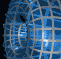Português | English Beyond 3D > Math Horizon > Mathematics

# The Mathematics of Math Horizon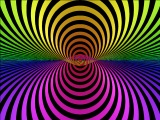"Math Horizon" is a view of a two-dimensional sphere immersed in four-space so that it has exactly one point of self-intersection. To see how this works, first note that surfaces in four-space generally intersect in points rather than in curves, as they do in three space. For example, if we label the axes x, y, z, and w, then the xy-plane and the zw-plane are two-dimensional planes in four space, but they intersect in only one point: the origin.

To form the sphere depicted in "Math Horizon", we began by taking the unit disc in the xy-plane and the unit disc in the wz-plane; since they intersect in a single point, these form the essential self-intersection in the surface. The trick now is to attach the boundaries of these two discs so as to form a sphere, and in such a way that no additional self intersection is produced.

The boundaries are two circles, which can be parameterized as (cos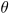, sin, 0, 0) and (0, 0, cos, sin). For a given, these two points, together with the origin, determine a plane in four-space (think of the points as vectors based at the origin that span the plane). For different values of, these planes intersect only at the origin, so if, for each, we connect the two boundary points by a curve lying in this plane, we will have joined the two disc boundaries to form a sphere with no additional self-intersection, as desired.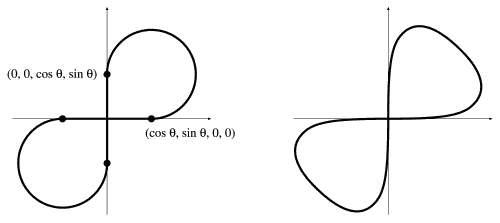The two points (cos, sin, 0, 0) and (0, 0, cos, sin) can be joined by circular arcs (left). A smooth figure-eight can replace the piecewise curve (right).

Note that the two points, when considered as vectors at the origin, are perpendicular unit vectors, so they act just like the unit x- and y-axes in the xy-plane. The intersection of the plane spanned by these vectors and one of the discs would be the segment from -1 to 1 along the x-axis, and with the other, the corresponding segment on the y-axis. These two segments form a "cross" at the origin, and one natural way to attach them is by two circular arcs thus forming a figure-8 with an axis of symmetry along the line y = x. A piecewise-defined version of the two-sphere in four-space can be produced in this way. On the other hand, we could form a smooth version of the surface if we had a smooth (rather than piecewise-defined) figure-8.

The equation (cos t, sin 2t) parameterizes a figure-8 that has the x-axis as an axis of symmetry, though the equation (cos t, (1/2)sin 2t) = (cos t, sin t cos t) = cos t (1, sin t) is more aesthetically pleasing, as the lobes of the figure-8 are rounder and cross at an angle of 90 degrees. Rotating this curve by 45 degrees about the origin produces a smooth figure-8 with its axis along the line y = x and its crossing tangent to the x and y axes, as desired. Using a standard rotation matrix with angle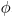=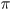/4, we obtain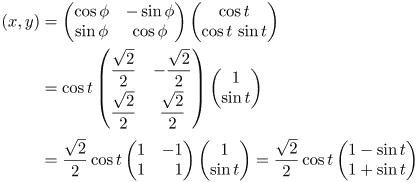Writing this in vector notation, we findNow, replacing the vectors (1,0) and (0,1) by the two vectors from the boundary of the discs in four-space gives a smooth parameterization by t andof the two-sphere in four-space with exactly one point of transverse self-intersection: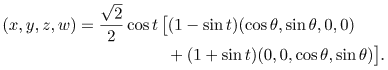Note that this surface lies within the unit sphere in four-space and touches the unit sphere when t = 0, namely along the curve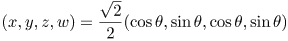a circle on the four-sphere. The image shown in "Math Horizons" is the stereographic projection of this surface from the point on this circle where= 0. Because the surface passes through the point of projection, it's image appears to extend out to infinity in three-space. Bands of the surface have been removed to help make the structure of the surface and its parameterization more apparent.